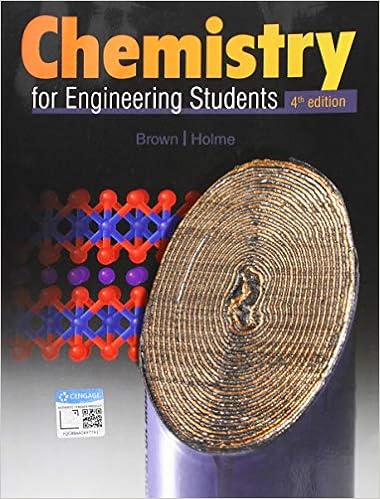# Pressure change in the flask without popping the

• Lab Report
• 8
• 91% (23) 21 out of 23 people found this document helpful

This preview shows page 5 - 8 out of 8 pages.

##### We have textbook solutions for you!
The document you are viewing contains questions related to this textbook.The document you are viewing contains questions related to this textbook.
Chapter 3 / Exercise 3.83
Chemistry for Engineering Students
Brown/HolmeExpert Verified
pressure change in the flask without popping the stopper. 4. The 3 M HCl need not be quantitatively prepared. 3.0 M HCl can be prepared by diluting 249 mL of 12 M HCl to a total volume of 1.0 liter. 5. The magnesium ribbon is the limiting reactant, thus the 5 mL of HCl does not have to be measured precisely. 6. For this experiment, you can use the syringe that is shipped with the Vernier Gas Pressure Sensor. When students are finished using the syringe, they should thoroughly clean it. Students will have to decide which pressure unit to use. They may benefit from a quick review regarding unit conversions. 7. Students will need access to a table of water vapor pressure at different temperatures. HAZARD ALERTS Hydrochloric Acid: Highly toxic by ingestion or inhalation; severely corrosive to skin and eyes. Hazard Code: A—Extremely hazardous. Magnesium, ribbon: Flammable solid; burns with an intense flame: Hazard Code: C—Somewhat hazardous. The hazard information reference is: Flinn Scientific, Inc., Chemical and Biological Catalog Reference Manual, P.O. Box 219, Batavia, IL 60510, (800) 452-1261, Adapted from Advanced Chemistry with Vernier & Laboratory Experiments for Advanced Placement Chemistry by Sally Ann Vonderbrink, Ph. D.
##### We have textbook solutions for you!
The document you are viewing contains questions related to this textbook.The document you are viewing contains questions related to this textbook.
Chapter 3 / Exercise 3.83
Chemistry for Engineering Students
Brown/HolmeExpert Verified
The Determination of the Molar Volume of a Gas SAMPLE DATA TABLE *Mass of Mg (g) 0.012 g Volume of flask (mL) 146.0 mL Maximum pressure (atm) 1.07 atm Initial pressure (atm) 0.991 atm Pressure change, ∆ P (atm) 0.079 atm Temperature (K) 296 K Vapor pressure of water (atm) 22.1 mm Hg = 0.02777 atm Answer to PRE-LAB QUESTION A reaction of 0.028 g of magnesium with excess hydrochloric acid generated 31.0 mL of gas. The gas was collected by water displacement in a 22 °C water bath. The barometric pressure that day was 746 mm Hg. (a) Use Dalton’s law and the vapor pressure of water at 22 °C (the table value is 19.8 mm Hg) to calculate the partial pressure of hydrogen gas collected.
(b) Use the combined law to calculate the “corrected” volume of hydrogen gas collected at STP.
(c) What is the theoretical number of moles of hydrogen that can be produced from 0.028 g of Mg? )
(d) Divide the corrected volume of hydrogen by the theoretical number of moles of hydrogen to calculate the molar volume (L/mol) of hydrogen at STP.
Adapted from Advanced Chemistry with Vernier & Laboratory Experiments for Advanced Placement Chemistry by Sally Ann Vonderbrink, Ph. D.
The Determination of the Molar Volume of a Gas Answers to POST-LAB QUESTIONS AND DATA ANALYSIS 1. *Calculate the mass of each piece of magnesium that you used if an analytical balance was unavailable.
•••